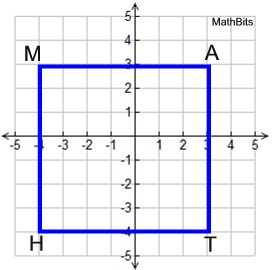Midpoint Practice Terms of Use    Contact Person: Donna RobertsDirections: Grab a paper and pencil to make your computations.

1.
Find the midpoint of the segment connecting the points (5,3) and (7,-2).
Choose:
 (1, -2½) (6, 1½) (6, ½) (1, ½)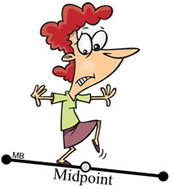2.
Find the midpoint of a segment connecting the points (a, b) and (4a, 3b).
Choose:
 (2a, 2b) (1.5a, 2b) (2.5a, b) (2.5a, 2b)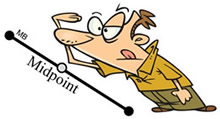3.
Given M is the midpoint of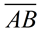. The coordinates of A are (-4,2) and the corrdinates of M are (1,1). Find the coordinates of B.
Choose:
 (-2, 4) (-2, 0) (6, 4) (6, 0)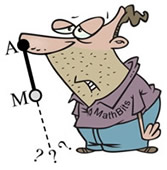4.
The coordinates of quadrilateral ABCD are A(1,3), B(7,5), C(3,2) and D(-3,-1).
a) Do the diagonals bisect each other?
Choose:
 Yes No

b)
What is the midpoint of the longer diagonal?
Choose:
 (2,2) (5,2) (2,1) (5,1)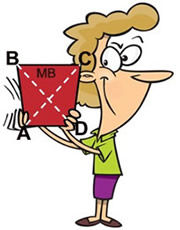5.
At what coordinates will a perpendicular bisector intersect withif A(-5,-3) and B(5,-8)?
Choose:
 (-5, -2.5) (0, -2.5) (-5, -5.5) (0, -5.5)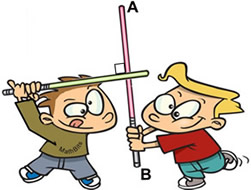6.
The ends of the diameter of a circle are located at (-4,-2) and (5,3). What are the coordinates of the center of the circle?
Choose:
 (0.5, 0.5) (-4.5, -2.5) (0.5, -2.5) (-4.5, 0.5)7.is the diameter of a circle whose center is the point (4,-3). If the coordinates of A are (-1,5), find the coordiantes of B.
Choose:
 (9, -9) (-6, 7) (9, -11) (-6, 13)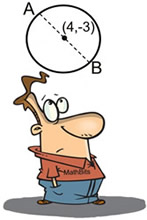8.
Square MATH is shown at the right. Assume its vertices have integer coordinates. What are the coordinates of the midpoint of the diagonal from H to A?
Choose:
 (3.5, 3.5) (-3.5, -3.5) (0.5, 0.5) (-0.5, -0.5)